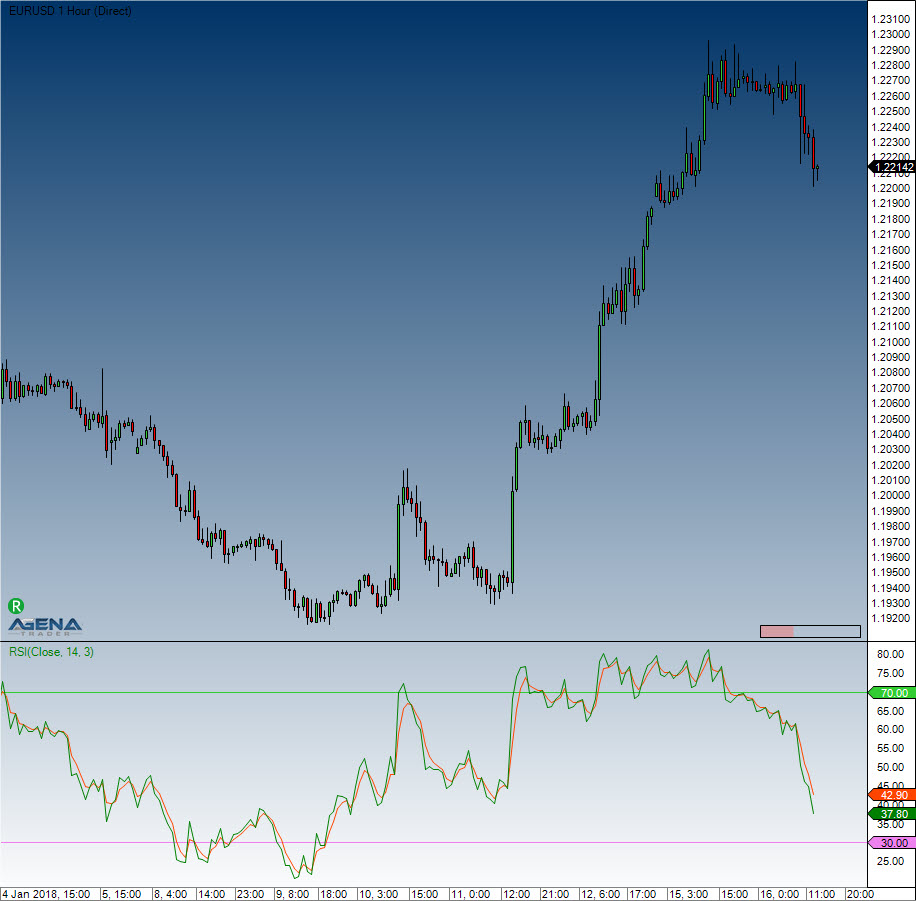# Relative Strength Index (RSI)

#### Description

J. Welles Wilder, the creator of several indicators, also developed the RSI, which is one of the most popular, useful momentum oscillators. It compares the size of the most recent profits with the size of the most recent losses, which means that it assesses the relationships between the up and down closing prices. The values are shown between 0 and 100.

#### Interpretation

Traditionally, and according to Wilder, RSI is considered overbought when above 70 and oversold when below 30. Signals can also be generated by searching for divergences, failure swings and centerline crossovers. RSI can also be used to identify the general trend. These traditional levels can also be adjusted to better fit the security or analytical requirements. Raising overbought to 80 or lowering oversold to 20 will reduce the number of overbought/oversold readings. Short-term traders sometimes use 2-period RSI to look for overbought readings above 80 and oversold readings below 20.

#### Usage

RSI(int period, int smooth)
RSI(IDataSeries inSeries, int period, int smooth)
RSI(int period, int smooth)[int barsAgo]
RSI(IDataSeries inSeries, int period, int smooth)[int barsAgo]
//For the average value
RSI(int period, int smooth).Avg[int barsAgo]
RSI(IDataSeries inSeries, int period, int smooth).Avg[int barsAgo]

#### Return value

double
When using this method with an index (e.g. RSI(14,3)[int barsAgo] ), the value of the indicator will be issued for the referenced bar.

#### Parameters

inSeries Input data series for the indicator
period Number of bars included in the calculations
smooth Number of periods for the smoothing

#### VisualizationRelative Strength Index (RSI)

#### Example

//Output for the RSI
Print("The current value for the RSI is: " + RSI(14, 3));
//Output for the average line
Print("The current value for the AVG line is: " + RSI(14, 3).Avg);